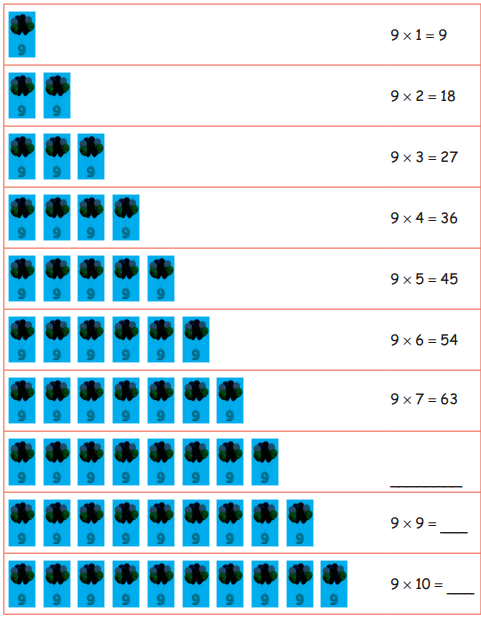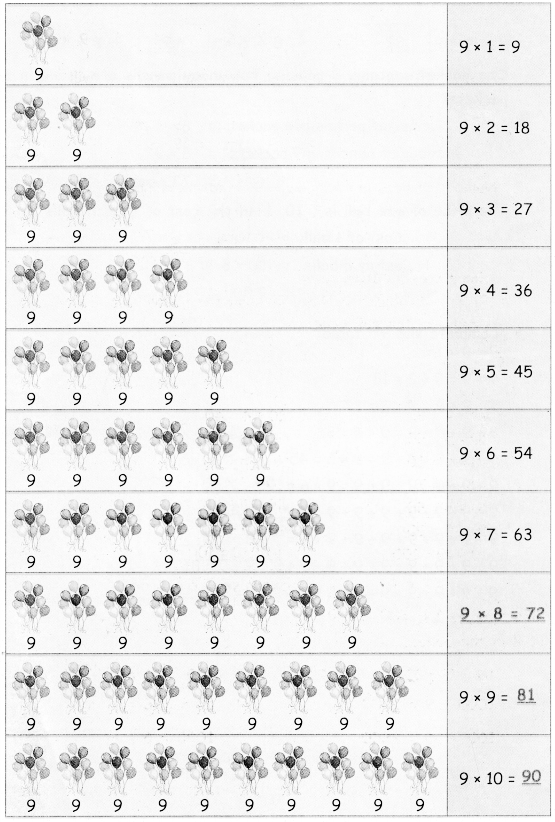Students can download 4th Maths Term 2 Chapter 2 Numbers Ex 2.4 Questions and Answers, Notes, Samacheer Kalvi 4th Maths Guide Pdf helps you to revise the complete Tamilnadu State Board New Syllabus, helps students complete homework assignments and to score high marks in board exams.

## Tamilnadu Samacheer Kalvi 4th Maths Solutions Term 2 Chapter 2 Numbers Ex 2.4

Question 1.
8 × 4 = ______
8 × 4 =   32Question 2.
8 × 6 = ______
8 × 6 =   48

Question 3.
8 ×10 = ______
8 × 10 =   80Question 4.
One packet contains 8 pencils, how many pencils are there in 9 such packets?
Number of pencils in a packet = 8
Number of pencils in 9 packets = 8 × 9
= 72 pencils

Question 5.
The price of one ball is 10. Find the cost of 8 such balls?
Cost of 1 ball = ₹ 10
∴ Cost of 8 balls = 10 × 8
= ₹ 80Complete the 9th tablre:
9 = 9 × 1 =
9 + 9 = 9 × 2 = 18
9 + 9 + 9 = 9 × 3 = 27
9 + 9 + 9 + 9 = 9 × 4 = 36
9 + 9 + 9 + 9 + 9 = 9 × 5 = 45
9 + 9 + 9 + 9 + 9 + 9 = 9 × 6 = ______
9 + 9 + 9 + 9 + 9 + 9 + 9 = 9 × 7 = ______
9 + 9 + 9 + 9 + 9 + 9 + 9 + 9 = 9 × 8 = ______
9 + 9 + 9 + 9 + 9 + 9 + 9 + 9 + 9 = 9 × 9 = 81
9 + 9 + 9 + 9 + 9 + 9 + 9 + 9 + 9 + 9 = 9 × 10 = ______
9 = 9 × 1 =
9 + 9 = 9 × 2 = 18
9 + 9 + 9 = 9 × 3 = 27
9 + 9 + 9 + 9 = 9 × 4 = 36
9 + 9 + 9 + 9 + 9 = 9 × 5 = 45
9 + 9 + 9 + 9 + 9 + 9 = 9 × 6 = 54
9 + 9 + 9 + 9 + 9 + 9 + 9 = 9 × 7 = 63
9 + 9 + 9 + 9 + 9 + 9 + 9 + 9 = 9 × 8 = 72
9 + 9 + 9 + 9 + 9 + 9 + 9 + 9 + 9 = 9 × 9 = 81
9 + 9 + 9 + 9 + 9 + 9 + 9 + 9 + 9 + 9 = 9 × 10 = 90